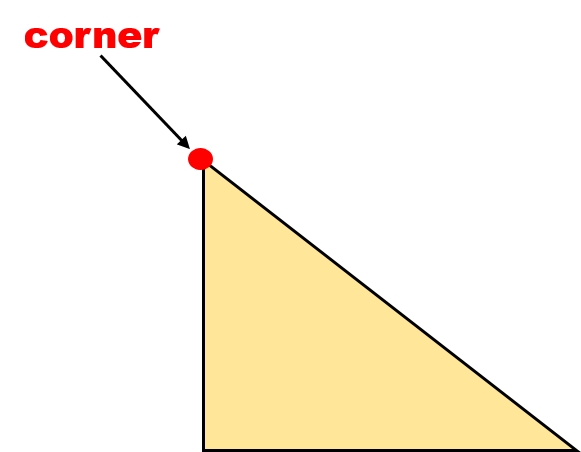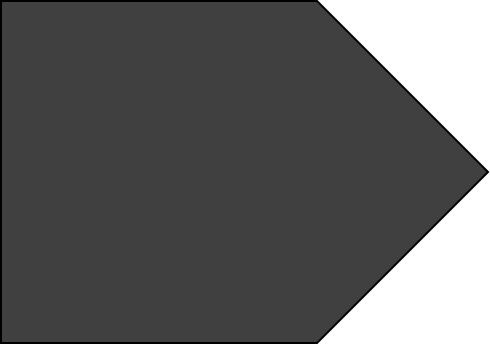1
visibility

Which of the following shapes has fewer corners?

• A• BThe correct answer is.

A corner (also called a vertex) is a point where two sides meet.

See example:We count the total number of corners.

There are 3 corners.

Solution:

Consider the shape below:We count the total number of corners.

There are 4 corners.

Consider the shape below:We count the total number of corners.

There are 5 corners.

Since, 4 < 5

So,has fewer corners.